asked on

# Power BI - DAX how to get the Average days between two dates?

Unable to solve the Average days issue using DAX. Here is the example.

## Average date between two dates in Power BI using DAX not working.

Is there a way to solve this using DAX?

? Average days between Registration Date and Start Date (For all user IDs the difference between Start Date minus Registration Date.

Power BILast Comment
SQL Power BI

8/22/2022 - Mon
Tom Farrar

Can you provide example answers for the 3 lines in you question?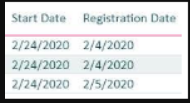SQL Power BI

I need the third column in the image about as "Number of Days" by calculation the Average of number of days between the Start date and Registration date for a given user id. What was the average number of days when it took a given user to register for a course and when they started the course.
Norie

Where's the ID column?
SQL Power BI

User id's are emails I can't share but assume that each user is taking a course or multiple users taking the same course. But I'm trying to find out the Average number of days using DAX based on when it took a given user to register for a course and when they started the course but I need the Average number of days as third column.

This is really urgent.
Thank you
SQL Power BI

I tried this but it didn't work.

Average Duration = AVERAGEX(‘Table1, DATEDIFF('Table1'[Registration Date], RELATED('Table2'[Start Date]), DAY))
SQL Power BI

Average Duration = AVERAGEX(‘Table1', DATEDIFF('Table1'[Registration Date], RELATED('Table2'[Start Date]), DAY))
SQL Power BI

Any ideas?
SQL Power BI

The DATEDIFF works on both the Registration Date and start Date but the AVERAGE Does not work.
Tom Farrar

How can there be an average for a given user id? For one user the difference is what it is?  That is why I asked for an example.
SQL Power BI

I just need the Average days between Registration Date and Start Date. Do you have some code in DAX etc to do that?
SQL Power BI

It looks like no Experts here to answer this question?
Tom Farrar

Are you trying to create a measure or a calculated column?  I think you want a measure, right?
SQL Power BI

Either works fine
Cédric Tielemans

seems this question is a duplicate.
Tom Farrar

Here is an example that works if I understand your requirement.
EEExample.pbix

SQL Power BI

@Tom

Your example gives the same result. Here is the result screen shot from your Power Bi File. I want the Average to show up in every row instead of Total at the end?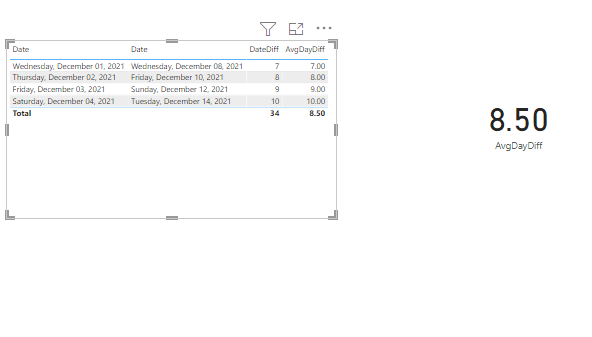Tom Farrar

You want the average 8.5 to show up in every row of the table, or report?  EEExample.pbix
SQL Power BI

Every row of the table.
Tom Farrar

Then you need to create a calculated column within the table.  Right click the table name and select "New Column".  Then add this code:

AvgDayDiff =
AVERAGEX(
All(Table1), DATEDIFF(Table1[Date], RELATED(Table2[Date]),DAY))

EEExample.pbix

SQL Power BI

When I click add Calculated Column it brings up the M Code Window. I'm not sure what other ways. Your code repeats same for all row but it should be different based on those different dates.

Tom Farrar

If you expect tp get a different answer for each row in the table, then that answer is simply subtracting one column from the other.  I added a column in the table that gives you the average of all the differences, and would not understand what you are looking for without a specific example showing the two columns (apparently in different tables) and the resultant column with the values you are expecting.
As for why you are getting the M code window, I expect you are in the Power Query functionality of Power BI.  That is all I can think of.
SQL Power BI

I also tried something like this but it does not return anything. I created a measure.

Avg # of Days =
``````DATEADD(    LASTDATE([Start Date]),    DATEDIFF(        LASTDATE([Start Date]),        LASTDATE([Registration Date]),        DAY    )/2,    DAY )
``````
SQL Power BI

How can I get the Avg # of days to show a digit instead of a whole date? Here is the screen shot and DAX

Duration = DATEDIFF('Table1'[Registration Date], RELATED('Table2'[Start Date]), DAY)

Average # of Days  = DATEADD(LASTDATE('Table1'[Registration Date]), DATEDIFF(LASTDATE( 'Table1'[Registration Date]),LASTDATE('Table2'[Start Date] ),DAY)/2,DAY)SQL Power BI

I also changed the Average # of Days Column to text, short date, date but I still get the whole date instead of a number as an Average. Any ideas?
SQL Power BI

Any Experts out there? Any ideas and suggestions?
SQL Power BI

Why the AVERAGEX provides the same result as DATEDIFF but not the Average number of Days?

Duration = DATEDIFF(Table1'[Registration Date], RELATED(‘Table2’[Start Date]), DAY)

Average # of Days  = AVERAGEX('Table1'[Registration Date]) - RELATED('Table2'[Start Date ])SQL Power BI

Tom Farrar

Never saw the screen shot.  Still would like to see a simple example...
Could try this formula---

Average Duration =
AVERAGEX(‘Table1,
'Table1'[Registration Date] - RELATED('Table2'[Start Date])
SQL Power BI

It gives the same values as Duration with DATEDIFF,

Duration = DATEDIFF(Table1'[Registration Date], RELATED(‘Table2’[Start Date]), DAY)
Average # of Days 10 =
AVERAGEX(‘Table1,
'Table1'[Registration Date] - RELATED('Table2'[Start Date])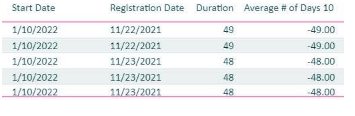Tom Farrar

What value are you expecting in the Duration column?

SQL Power BI

Duration column values are correct but not the Average # of Days 10. The following is not returning for every row but total. Also why it's the same value as duration?

Average # of Days 10 =
AVERAGEX(‘Table1,
'Table1'[Registration Date] - RELATED('Table2'[Start Date])
Tom Farrar

Where are you creating the formula?  Are you creating a measure for it?

SQL Power BI

Yes I'm creating a measure.
Tom Farrar

You said:
"Duration column values are correct but not the Average # of Days 10. The following is not returning for every row but total. Also why it's the same value as duration?

Average # of Days 10 =
AVERAGEX(‘Table1,
'Table1'[Registration Date] - RELATED('Table2'[Start Date])"

My question again is:
In the example data you provided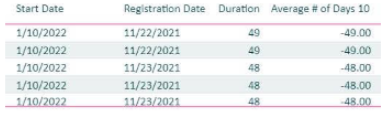What should the answer be in Average # of Days 10?  And what is the significance of 10?

SQL Power BI

There is no significance of 10 but it's just a measure name as I have created many measures with name 1, 2 3 and so on.
The answer for the Average # of Days 10  should be  =  The average difference between Registration Date and Start Date. So for all users the difference between the Start Date minus the Registration Date. So every row in the screen shot should show a number displaying the average difference between the Start date minus the Registration Date. Please don't look at the duration which is correct but I can't figure out the Average difference between the start date minus the registration date?
Any ideas and codes to fix this? It has been taking long time and no experts here can help.
Tom Farrar

I look back through our comments and it feels like we are experiencing "Ground Hog Day".  If you look at each line in your example the duration is the average, the average of one row.  If you think it should be a different number, then like I have asked before, what should that numbers be in your example?  What should show up for each line below?SQL Power BI

Duration is not the Average. It's the difference between two date. Here is the DAX for that.
Duration = DATEDIFF(Table1'[Registration Date], RELATED(‘Table2’[Start Date]), DAY)

But I'm looking for an average between those two dates. I don't know exactly how each rows values but it should give me the average difference of those two dates. Let's base it on users who took those courses and what was the average of those two dates when the user registered in the course and when it started. IT should show for each row the average of those dates. Any ideas?
Tom Farrar

So, back to my original question based on the data in your example:

What values would you expect to see for each row instead of 49?
SQL Power BI

I don't really know what values can be there for each row for the Average. But I was thinking it should be different than the duration which is the date difference but not the average. I know you calculate Average by summing the numbers and divide by the total number but in the case I'm really lost.
Tom Farrar

Well the average for the registrations on the 22nd and starting on the 10th is 49 (49+49)/2.  That is what your data shows. If one of the examples had registered on the 22nd, but started on the 20th, then the average would be 59 (49+59)/2.  If you had 10 students registering on the 22nd, and each of them started on different dates, then the average would be the 10 durations added together and divided by 10.

Now if you want the average for all registrations and start dates from your example, then it would be 48.4 (49+49+48+48+48)/5.  But you would get that for all the rows.

A measure is an aggregation, and is not meant to be a value line-by-line in the rows of the data.
SQL Power BI

Thanks for the explanation. I know measure is an aggregation and in the total it shows correct average. But I tried calculated column but still same issue? Here is the result again. How can this be achieved row by row instead of showing Average at the bottom on the total.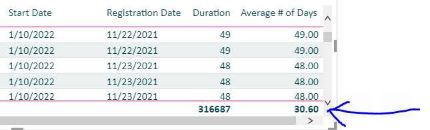Tom Farrar

I answered that question in an earlier comment (Dec 10th).  See the formula below.  I believe this will get you the total average on each line.
Generally when the total average is calculated like this it is an interim calculation.  The result is used to calculate a percentage or difference from the actual.

AvgDayDiff =
AVERAGEX(
All(Table1), DATEDIFF(Table1[Date], RELATED(Table2[Date]),DAY))
SQL Power BI

Great. Let me try and see if it works

Thank you so much for your help.
SQL Power BI

Can I use a measure or Calculated Column for calculating the Average?
Tom Farrar

Generally I think you can use either if you calculate as shown in the formula.  I think you will have more luck with a measure for reporting purposes.  Not sure if you are going to use the total average to build percentages or differences from actual, or...?
SQL Power BI

Your formula is generating the same value for all rows. There are thousands of rows with same number such as 24.84 as shown in the screen shot. It's the last column. I created both measure and Calculated column.

Average # of Days 14 =
AVERAGEX(
All(Table1), DATEDIFF(Table1[Date], RELATED(Table2[Date]),DAY))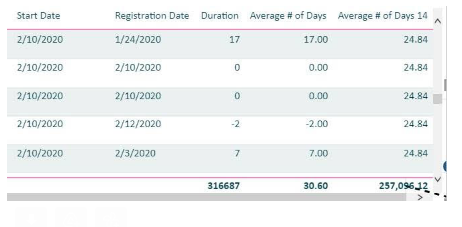Tom Farrar

What are you trying to achieve?  How are you trying to analyze the data?  Do you want to know the average time it took from Registration to Start?  Then take the Start Date out of the report.  If you want to know the average number of days for female and male registration to start then take out the dates from the report and put in the gender column (if there is one).

If you put both dates in the report, your average is going to be the same as the duration.
SQL Power BI

Yes. I want to know the average time it took from Registration to Start?
Tom Farrar

At what level of aggregation?  If you look at each occurrence, then the average will be the same as the duration.  If you look at an aggregation that has the same Registration Date and Start Date as the other occurrences in the aggregation, then the average is going to be the same as the duration.  It is only when you aggregate occurrences that have different Registration Dates and/or Start Dates that the average is going to be different from the duration.  As far as I am concerned, the AverageX function provided earlier is what you are needing, unless you can explain (with specific examples) what you expect the average to be for occurrences.
SQL Power BI

Thanks for the explanation. Yes there are different Start Dates and Registration dates and I want the Average to be different than the Duration but why it's the same?
Tom Farrar

The average and duration will be different for any aggregation of different Start Dates and Registration Dates.  Show meaan example where this is not the case.
SQL Power BI

I don't understand why the Average and Duration are always same. They should be different by looking at the formulas. Here is a result and as you can see the Start date and Registration date are different but the Duration and Average columns have the same value for each row. I'm really running out of time ad ideas. Any ideas?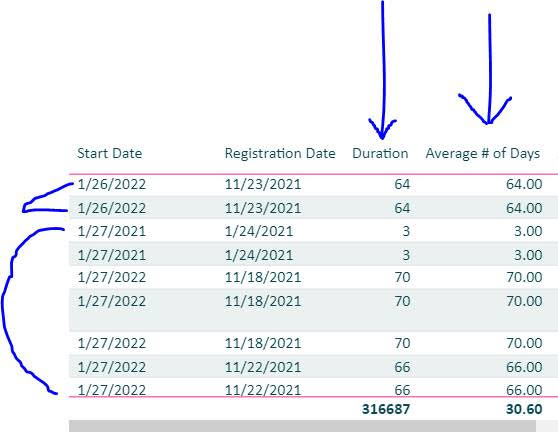SQL Power BI

How to solve the above?
For all user id's the difference between the start date minus the registration date?
Lets say you have user id1, user id2 and so on.
Tom Farrar

You can't put the start date, registration date and duration in the visual together.  The duration will be the same as the average for each row.  Take the dates and duration out of the visual and put in the UserID (assuming a user has registered and started multiple classes that he/she registered and started on different times), then you will see a different average.
SQL Power BI

I did that and just using the Average in the screen shot below but it shows the datediiff for duration instead of AverageX. User id is hidden in this screen shot. If you look at the dates for average it's showing the DateDiff instead of Average here. I just have to be 100% sure it's calculating the Average before deploying the report for business users but not the DateDiff. How can you verify mathematically the average is correct between the start date and registration date by looking at the result below? Please help.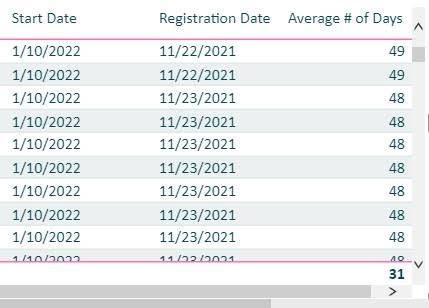Tom Farrar

The average measure result is filter context, that means if you include the dates in the visual your duration and average will be the same.  Show me the same visual without the dates, and including the User ID only and the Average # Of Days.
SQL Power BI

Ok. I can not show user id's here but the user id's are repeating in the visual so one user can have registration in multiple courses and the average number is repeating.. But I need to prove it to the report consumers the the average is correct but not sure how?
Tom Farrar

What are you including the report visual besides the User ID and Average?
SQL Power BI

Ok so what I did was first I exported the visual to Excel with all those columns and then removed all those column but only included user id and Average # of Days columns in Excel and exported them. Then I looked at one user id which was repeating 5 times in the first visual with all those columns including start date and registration date. So I looked at all those 5 values  for dates and average numbers for that user and summed them up and used Average Formula and I got 55 which is correct. But now how I can display that user id just one time in the visual to get the 55 as Average for example?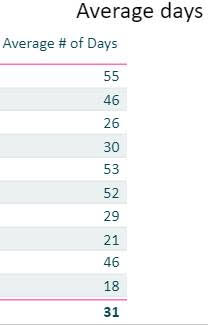Tom Farrar

The problem is you are using is a calculated column if the data resides in the table.  You need to create a measure.  It will aggregate the data by user in the report and give you a consolidated average.

In the Power BI visual go to the table where the data resides, right-click and select "New Measure".  Then post the formula you have for the average in as the new measure.  It will show up under the table in the view, and add that to your visual and take out the one that came from the table (the calculated column).
SQL Power BI

I'm using a measure and 100% sure.
Tom Farrar

Not if I understand this statement:

"included user id and Average # of Days columns in Excel and exported them "
SQL Power BI

Here is the measure DAX I'm using for the Average # of Days.
Measure  = AVERAGEX(‘Table 1', DATEDIFF(‘Table 1’[Registration Date], RELATED(‘Table 2’[Start Date]), DAY))
Tom Farrar

Where does that formula reside?
SQL Power BI

The user id's are repeating and I found that the average is correct when I exported the visual in Excel and looked at the numbers. For one user there were 5 rows of different averages and I used the Average formula in Excel and I got the correct result. But how I can I fix the repeating user id's so I only get one row per user and one average number in the visual?
SQL Power BI